1 Units and Measurement

1.7 Solving Problems in Physics

Learning Objectives

By the end of this section, you will be able to:

• Describe the process for developing a problem-solving strategy.
• Explain how to find the numerical solution to a problem.
• Summarize the process for assessing the significance of the numerical solution to a problem.

Problem-solving skills are clearly essential to success in a quantitative course in physics. More important, the ability to apply broad physical principles—usually represented by equations—to specific situations is a very powerful form of knowledge. It is much more powerful than memorizing a list of facts. Analytical skills and problem-solving abilities can be applied to new situations whereas a list of facts cannot be made long enough to contain every possible circumstance. Such analytical skills are useful both for solving problems in this text and for applying physics in everyday life.

As you are probably well aware, a certain amount of creativity and insight is required to solve problems. No rigid procedure works every time. Creativity and insight grow with experience. With practice, the basics of problem solving become almost automatic. One way to get practice is to work out the text’s examples for yourself as you read. Another is to work as many end-of-section problems as possible, starting with the easiest to build confidence and then progressing to the more difficult. After you become involved in physics, you will see it all around you, and you can begin to apply it to situations you encounter outside the classroom, just as is done in many of the applications in this text.

Although there is no simple step-by-step method that works for every problem, the following three-stage process facilitates problem solving and makes it more meaningful. The three stages are strategy, solution, and significance. This process is used in examples throughout the book. Here, we look at each stage of the process in turn.

Strategy

Strategy is the beginning stage of solving a problem. The idea is to figure out exactly what the problem is and then develop a strategy for solving it. Some general advice for this stage is as follows:

• Examine the situation to determine which physical principles are involved. It often helps to draw a simple sketch at the outset. You often need to decide which direction is positive and note that on your sketch. When you have identified the physical principles, it is much easier to find and apply the equations representing those principles. Although finding the correct equation is essential, keep in mind that equations represent physical principles, laws of nature, and relationships among physical quantities. Without a conceptual understanding of a problem, a numerical solution is meaningless.
• Make a list of what is given or can be inferred from the problem as stated (identify the “knowns”). Many problems are stated very succinctly and require some inspection to determine what is known. Drawing a sketch can be very useful at this point as well. Formally identifying the knowns is of particular importance in applying physics to real-world situations. For example, the word stopped means the velocity is zero at that instant. Also, we can often take initial time and position as zero by the appropriate choice of coordinate system.
• Identify exactly what needs to be determined in the problem (identify the unknowns). In complex problems, especially, it is not always obvious what needs to be found or in what sequence. Making a list can help identify the unknowns.
• Determine which physical principles can help you solve the problem. Since physical principles tend to be expressed in the form of mathematical equations, a list of knowns and unknowns can help here. It is easiest if you can find equations that contain only one unknown—that is, all the other variables are known—so you can solve for the unknown easily. If the equation contains more than one unknown, then additional equations are needed to solve the problem. In some problems, several unknowns must be determined to get at the one needed most. In such problems it is especially important to keep physical principles in mind to avoid going astray in a sea of equations. You may have to use two (or more) different equations to get the final answer.

Solution

The solution stage is when you do the math. Substitute the knowns (along with their units) into the appropriate equation and obtain numerical solutions complete with units. That is, do the algebra, calculus, geometry, or arithmetic necessary to find the unknown from the knowns, being sure to carry the units through the calculations. This step is clearly important because it produces the numerical answer, along with its units. Notice, however, that this stage is only one-third of the overall problem-solving process.

Significance

After having done the math in the solution stage of problem solving, it is tempting to think you are done. But, always remember that physics is not math. Rather, in doing physics, we use mathematics as a tool to help us understand nature. So, after you obtain a numerical answer, you should always assess its significance:

• Check your units. If the units of the answer are incorrect, then an error has been made and you should go back over your previous steps to find it. One way to find the mistake is to check all the equations you derived for dimensional consistency. However, be warned that correct units do not guarantee the numerical part of the answer is also correct.
• Check the answer to see whether it is reasonable. Does it make sense? This step is extremely important: –the goal of physics is to describe nature accurately. To determine whether the answer is reasonable, check both its magnitude and its sign, in addition to its units. The magnitude should be consistent with a rough estimate of what it should be. It should also compare reasonably with magnitudes of other quantities of the same type. The sign usually tells you about direction and should be consistent with your prior expectations. Your judgment will improve as you solve more physics problems, and it will become possible for you to make finer judgments regarding whether nature is described adequately by the answer to a problem. This step brings the problem back to its conceptual meaning. If you can judge whether the answer is reasonable, you have a deeper understanding of physics than just being able to solve a problem mechanically.
• Check to see whether the answer tells you something interesting. What does it mean? This is the flip side of the question: Does it make sense? Ultimately, physics is about understanding nature, and we solve physics problems to learn a little something about how nature operates. Therefore, assuming the answer does make sense, you should always take a moment to see if it tells you something about the world that you find interesting. Even if the answer to this particular problem is not very interesting to you, what about the method you used to solve it? Could the method be adapted to answer a question that you do find interesting? In many ways, it is in answering questions such as these that science progresses.

Summary

The three stages of the process for solving physics problems used in this book are as follows:

• Strategy: Determine which physical principles are involved and develop a strategy for using them to solve the problem.
• Solution: Do the math necessary to obtain a numerical solution complete with units.
• Significance: Check the solution to make sure it makes sense (correct units, reasonable magnitude and sign) and assess its significance.

Conceptual Questions

What information do you need to choose which equation or equations to use to solve a problem?

What should you do after obtaining a numerical answer when solving a problem?

Check to make sure it makes sense and assess its significance.

Consider the equation y = mt +b, where the dimension of y is length and the dimension of t is time, and m and b are constants. What are the dimensions and SI units of (a) m and (b) b?

Consider the equation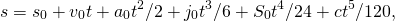where s is a length and t is a time. What are the dimensions and SI units of (a)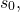(b)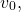(c)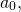(d)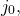(e)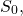and (f) c?

a.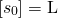and units are meters (m); b.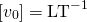and units are meters per second (m/s); c.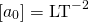and units are meters per second squared (m/s2); d.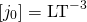and units are meters per second cubed (m/s3); e.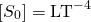and units are m/s4; f.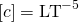and units are m/s5.

(a) A car speedometer has a 5% uncertainty. What is the range of possible speeds when it reads 90 km/h? (b) Convert this range to miles per hour. Note 1 km = 0.6214 mi.

A marathon runner completes a 42.188-km course in 2 h, 30 min, and 12 s. There is an uncertainty of 25 m in the distance traveled and an uncertainty of 1 s in the elapsed time. (a) Calculate the percent uncertainty in the distance. (b) Calculate the percent uncertainty in the elapsed time. (c) What is the average speed in meters per second? (d) What is the uncertainty in the average speed?

a. 0.059%; b. 0.01%; c. 4.681 m/s; d. 0.07%, 0.003 m/s

The sides of a small rectangular box are measured to be 1.80 ± 0.1 cm, 2.05 ± 0.02 cm, and 3.1 ± 0.1 cm long. Calculate its volume and uncertainty in cubic centimeters.

When nonmetric units were used in the United Kingdom, a unit of mass called the pound-mass (lbm) was used, where 1 lbm = 0.4539 kg. (a) If there is an uncertainty of 0.0001 kg in the pound-mass unit, what is its percent uncertainty? (b) Based on that percent uncertainty, what mass in pound-mass has an uncertainty of 1 kg when converted to kilograms?

a. 0.02%; b. 1×104 lbm

The length and width of a rectangular room are measured to be 3.955 ± 0.005 m and 3.050 ± 0.005 m. Calculate the area of the room and its uncertainty in square meters.

A car engine moves a piston with a circular cross-section of 7.500 ± 0.002 cm in diameter a distance of 3.250 ± 0.001 cm to compress the gas in the cylinder. (a) By what amount is the gas decreased in volume in cubic centimeters? (b) Find the uncertainty in this volume.

a. 143.6 cm3; b. 0.2 cm3 or 0.14%

Challenge Problems

The first atomic bomb was detonated on July 16, 1945, at the Trinity test site about 200 mi south of Los Alamos. In 1947, the U.S. government declassified a film reel of the explosion. From this film reel, British physicist G. I. Taylor was able to determine the rate at which the radius of the fireball from the blast grew. Using dimensional analysis, he was then able to deduce the amount of energy released in the explosion, which was a closely guarded secret at the time. Because of this, Taylor did not publish his results until 1950. This problem challenges you to recreate this famous calculation. (a) Using keen physical insight developed from years of experience, Taylor decided the radius r of the fireball should depend only on time since the explosion, t, the density of the air,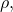and the energy of the initial explosion, E. Thus, he made the educated guess that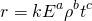for some dimensionless constant k and some unknown exponents a, b, and c. Given that [E] = ML2T–2, determine the values of the exponents necessary to make this equation dimensionally consistent. (Hint: Notice the equation implies that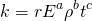and that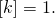) (b) By analyzing data from high-energy conventional explosives, Taylor found the formula he derived seemed to be valid as long as the constant k had the value 1.03. From the film reel, he was able to determine many values of r and the corresponding values of t. For example, he found that after 25.0 ms, the fireball had a radius of 130.0 m. Use these values, along with an average air density of 1.25 kg/m3, to calculate the initial energy release of the Trinity detonation in joules (J). (Hint: To get energy in joules, you need to make sure all the numbers you substitute in are expressed in terms of SI base units.) (c) The energy released in large explosions is often cited in units of “tons of TNT” (abbreviated “t TNT”), where 1 t TNT is about 4.2 GJ. Convert your answer to (b) into kilotons of TNT (that is, kt TNT). Compare your answer with the quick-and-dirty estimate of 10 kt TNT made by physicist Enrico Fermi shortly after witnessing the explosion from what was thought to be a safe distance. (Reportedly, Fermi made his estimate by dropping some shredded bits of paper right before the remnants of the shock wave hit him and looked to see how far they were carried by it.)

The purpose of this problem is to show the entire concept of dimensional consistency can be summarized by the old saying “You can’t add apples and oranges.” If you have studied power series expansions in a calculus course, you know the standard mathematical functions such as trigonometric functions, logarithms, and exponential functions can be expressed as infinite sums of the formwhere the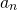are dimensionless constants for all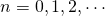and x is the argument of the function. (If you have not studied power series in calculus yet, just trust us.) Use this fact to explain why the requirement that all terms in an equation have the same dimensions is sufficient as a definition of dimensional consistency. That is, it actually implies the arguments of standard mathematical functions must be dimensionless, so it is not really necessary to make this latter condition a separate requirement of the definition of dimensional consistency as we have done in this section.